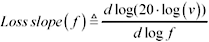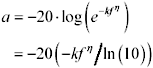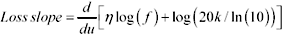# Appendix B. Calculation of Loss Slope

The loss slope, for the purposes of this discussion, is defined as the slope, using a log-log plot, of attenuation a , in dB, versus frequency f , in Hz.

Equation B.1Notice that since a , in dB, is already the logarithm of signal amplitude v in Volts, the loss-slope definition is making use of a double-logarithm. The next equation shows the relation between loss slope and signal amplitude.

Equation B.2As an example, let's determine the loss slope for a signal amplitude v that varies exponentially with some power h of frequency.

Equation B.3The attenuation a in dB is base-10-logarithmically related to the signal amplitude.

Equation B.4Substitute [B.3] for v , and simplify using the relation log( e x ) = x /ln(10).

Equation B.5And the log of attenuation is what we display in the loss-slope graph.

Equation B.6The loss slope is defined as d (log a )/ d (log f ). Let u = log f , and evaluate the loss slope as d (log a )/ du using [B.6] for the definition of log a .

Equation B.7Inside the square brackets the right-hand term remains constant and so contributes nothing to the derivative. In the left-hand term the expression log( f ) may be changed to u , producing this:

Equation B.8In conclusion, given a linear system whose attenuation in dB varies with f h , the loss slope is h .

In the skin-effect limited region the transmission-line attenuation in dB varies with the square root of f, producing a loss slope of 1/2. In the dielectric-loss region the attenuation varies directly with f, producing a loss slope of 1.

In a dispersion-limited transmission system the loss slope indicates the severity of the tradeoff between transmission distance and operating speed.High-Speed Signal Propagation[c] Advanced Black Magic
ISBN: 013084408X
EAN: N/A
Year: 2005
Pages: 163